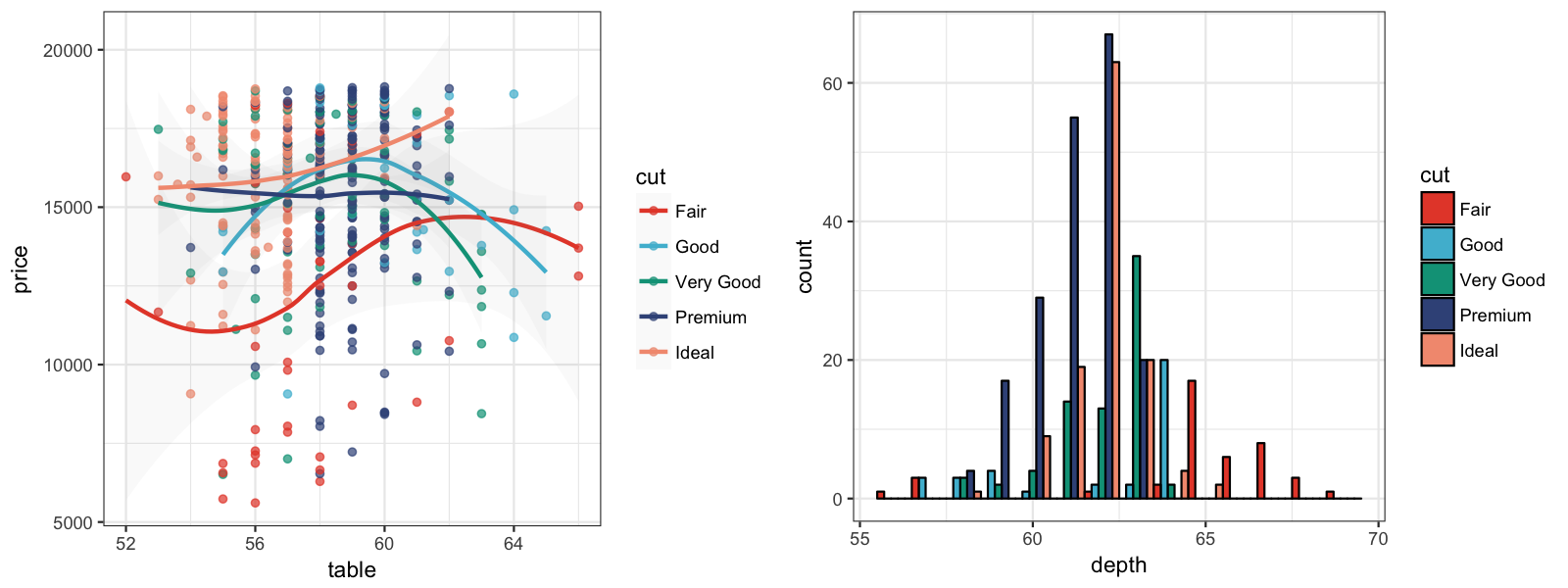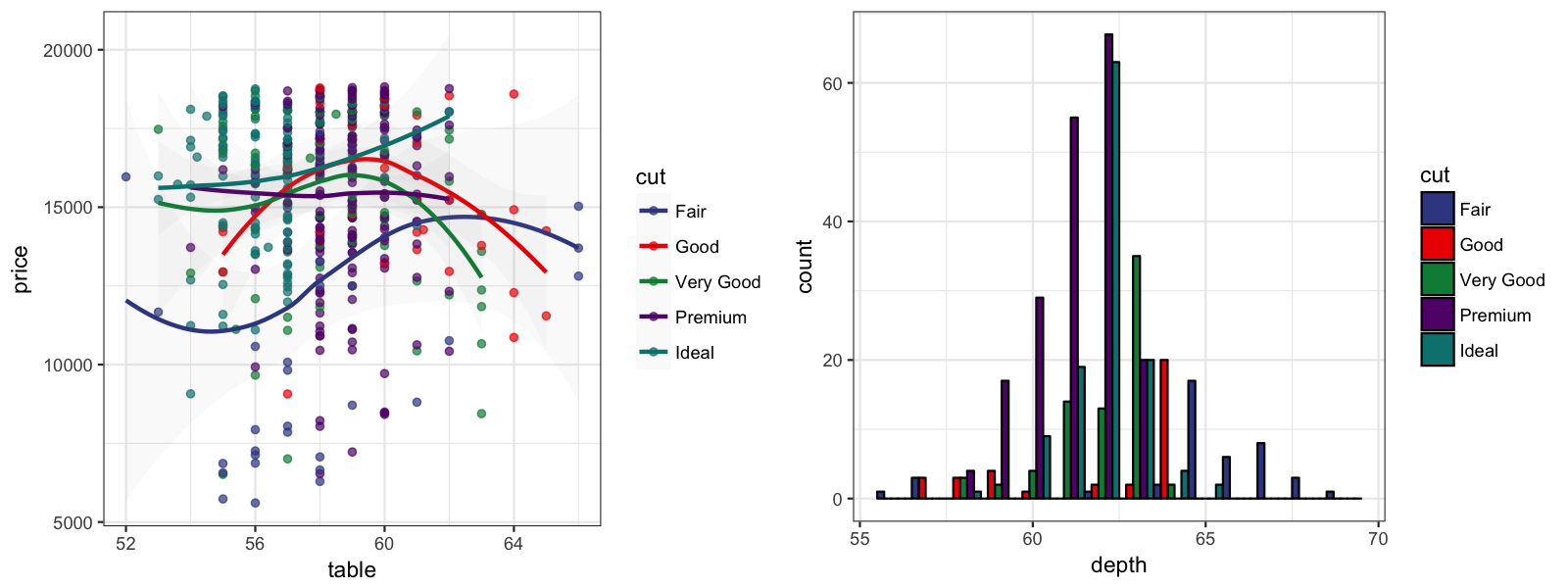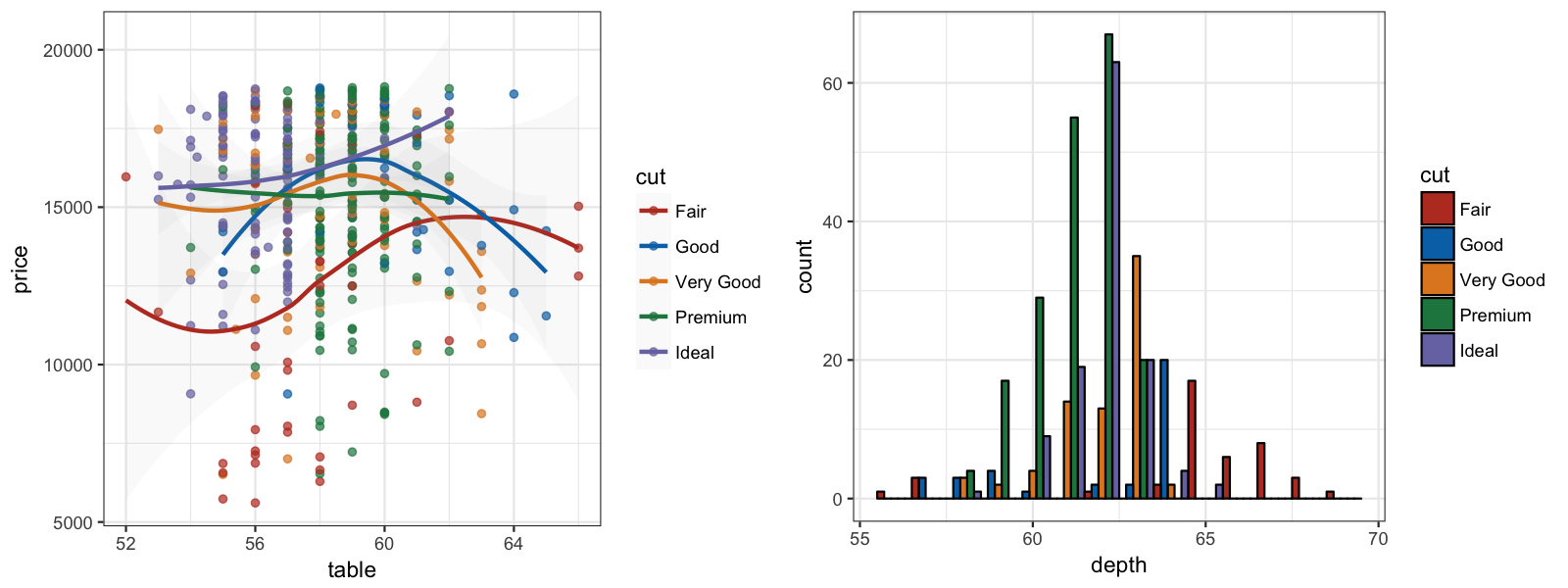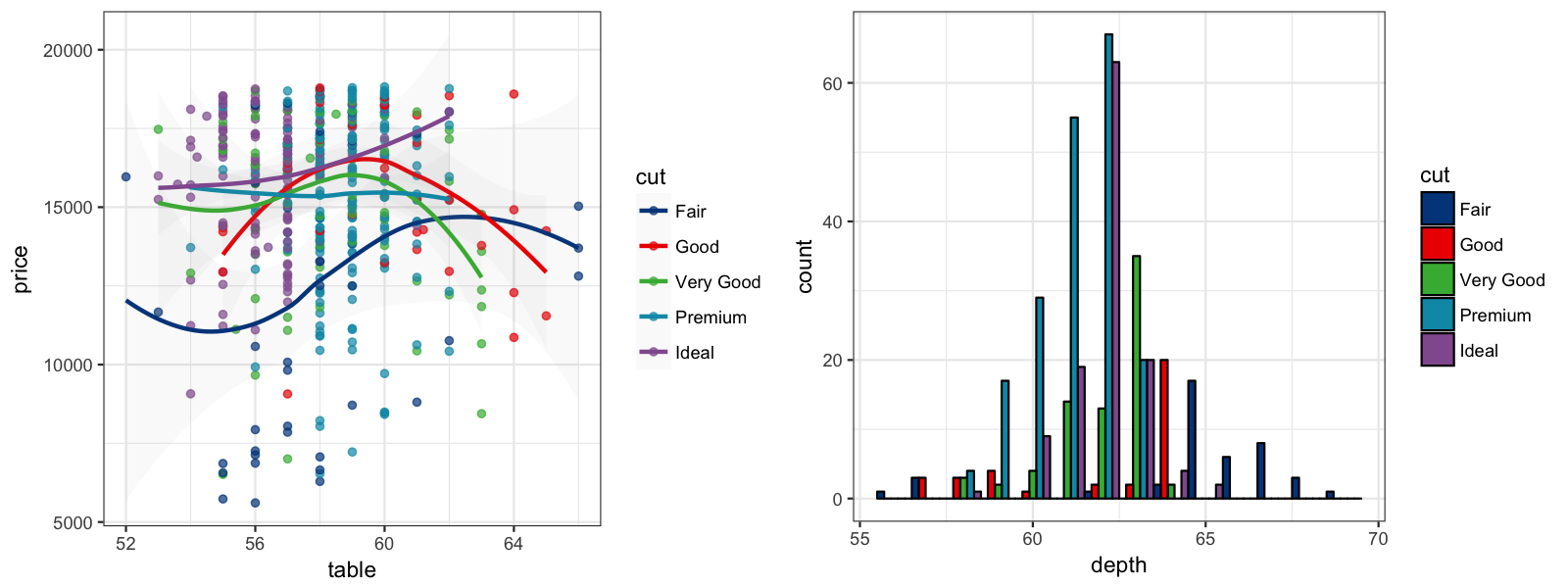# 1 Introduction

My eyes were finally opened and I understood nature.

I learned at the same time to love it.

— Claude Monet

`ggsci` offers a collection of high-quality color palettes inspired by colors used in scientific journals, data visualization libraries, science fiction movies, and TV shows. The color palettes in `ggsci` are available as `ggplot2` scales. For all the color palettes, the corresponding scales are named as:

• `scale_color_palname()`
• `scale_fill_palname()`

We also provided aliases, such as `scale_colour_palname()` for `scale_color_palname()`. All available color palettes are summarized in the table below.

Name Scales Palette Types Palette Generator

NPG

`scale_color_npg()` `scale_fill_npg()`

`"nrc"`

`pal_npg()`

AAAS

`scale_color_aaas()` `scale_fill_aaas()`

`"default"`

`pal_aaas()`

NEJM

`scale_color_nejm()` `scale_fill_nejm()`

`"default"`

`pal_nejm()`

Lancet

`scale_color_lancet()` `scale_fill_lancet()`

`"lanonc"`

`pal_lancet()`

JAMA

`scale_color_jama()` `scale_fill_jama()`

`"default"`

`pal_jama()`

JCO

`scale_color_jco()` `scale_fill_jco()`

`"default"`

`pal_jco()`

UCSCGB

`scale_color_ucscgb()` `scale_fill_ucscgb()`

`"default"`

`pal_ucscgb()`

D3

`scale_color_d3()`
`scale_fill_d3()`

`"category10"` `"category20"` `"category20b"` `"category20c"`

`pal_d3()`

LocusZoom

`scale_color_locuszoom()` `scale_fill_locuszoom()`

`"default"`

`pal_locuszoom()`

IGV

`scale_color_igv()` `scale_fill_igv()`

`"default"`
`"alternating"`

`pal_igv()`

UChicago

`scale_color_uchicago()` `scale_fill_uchicago()`

`"default"`
`"light"`
`"dark"`

`pal_uchicago()`

Star Trek

`scale_color_startrek()` `scale_fill_startrek()`

`"uniform"`

`pal_startrek()`

Tron Legacy

`scale_color_tron()` `scale_fill_tron()`

`"legacy"`

`pal_tron()`

Futurama

`scale_color_futurama()` `scale_fill_futurama()`

`"planetexpress"`

`pal_futurama()`

Rick and Morty

`scale_color_rickandmorty()` `scale_fill_rickandmorty()`

`"schwifty"`

`pal_rickandmorty()`

The Simpsons

`scale_color_simpsons()` `scale_fill_simpsons()`

`"springfield"`

`pal_simpsons()`

GSEA

`scale_color_gsea()` `scale_fill_gsea()`

`"default"`

`pal_gsea()`

Material Design

`scale_color_material()` `scale_fill_material()`

`"red"` `"pink"`
`"purple"` `"deep-purple"`
`"indigo"` `"blue"`
`"light-blue"` `"cyan"`
`"teal"` `"green"`
`"light-green"` `"lime"`
`"yellow"` `"amber"`
`"orange"` `"deep-orange"`
`"brown"` `"grey"`
`"blue-grey"`

`pal_material()`

# 2 Discrete Color Palettes

We will use scatterplots with smooth curves, and bar plots to demonstrate the discrete color palettes in `ggsci`.

``````library("ggsci")
library("ggplot2")
library("gridExtra")

data("diamonds")

p1 = ggplot(subset(diamonds, carat >= 2.2),
aes(x = table, y = price, colour = cut)) +
geom_point(alpha = 0.7) +
geom_smooth(method = "loess", alpha = 0.05, size = 1, span = 1) +
theme_bw()

p2 = ggplot(subset(diamonds, carat > 2.2 & depth > 55 & depth < 70),
aes(x = depth, fill = cut)) +
geom_histogram(colour = "black", binwidth = 1, position = "dodge") +
theme_bw()``````

## 2.1 NPG

The NPG palette is inspired by the plots in the journals published by Nature Publishing Group:

``````p1_npg = p1 + scale_color_npg()
p2_npg = p2 + scale_fill_npg()
grid.arrange(p1_npg, p2_npg, ncol = 2)``````## 2.2 AAAS

The AAAS palette is inspired by the plots in the journals published by American Association for the Advancement of Science:

``````p1_aaas = p1 + scale_color_aaas()
p2_aaas = p2 + scale_fill_aaas()
grid.arrange(p1_aaas, p2_aaas, ncol = 2)``````## 2.3 NEJM

The NEJM palette is inspired by the plots in The New England Journal of Medicine:

``````p1_nejm = p1 + scale_color_nejm()
p2_nejm = p2 + scale_fill_nejm()
grid.arrange(p1_nejm, p2_nejm, ncol = 2)``````## 2.4 Lancet

The Lancet palette is inspired by the plots in Lancet journals, such as Lancet Oncology:

``````p1_lancet = p1 + scale_color_lancet()
p2_lancet = p2 + scale_fill_lancet()
grid.arrange(p1_lancet, p2_lancet, ncol = 2)``````## 2.5 JAMA

The JAMA palette is inspired by the plots in The Journal of the American Medical Association:

``````p1_jama = p1 + scale_color_jama()
p2_jama = p2 + scale_fill_jama()
grid.arrange(p1_jama, p2_jama, ncol = 2)``````